# Grade - math word problems

#### Number of problems found: 5415

• Cross sectionThe cross-section ABCD of a swimming pool is a trapezium. Its width AB=14 meters, depth at the shallow end is 1.5 meters, and at the deep end is 8 meters. Find the area of the cross-section.
• Iron pole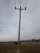The iron pole is in the ground 2/5 of its length, partly above the ground 1/3 is yellow, and the unpainted section is 6 m long. How long is the entire column?
• Forest nurseryIn the forest nursery after winter, they found that 1/10 stems died out of them. For them, they land 193 new spruces. How many spruces are in the forest nursery?
• InscribedCube is inscribed in the cube. Determine its volume if the edge of the cube is 10 cm long.
• Double 5Peter was thinking of a number. Peter doubles it and gets an answer of 8.6. What was the original number?
• Number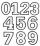Which number is 17 times larger than the number 6?
• RemainderA is an arbitrary integer that gives remainder 1 in the division with 6. B is an arbitrary integer that gives remainder 2 the division by. What makes remainder in division by 3 product of numbers A x B ?
• Touch x-axisFind the equations of circles that pass through points A (-2; 4) and B (0; 2) and touch the x-axis.
• Horizontal distance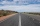The road has a gradient of 8%. How many meters will the road rise on a horizontal distance of 400m?
• Bricks wallThere are 5000 bricks. How high wall thickness of 20 cm around the area which has dimensions 20 m and 15 m can use these bricks to build? Brick dimensions are 30 cm, 20 cm and 10 cm.Find the roots of the quadratic equation: 3x2-4x + (-4) = 0.
• Average ageThe average age of all people at the celebration was equal to the number of people present. After the departure of one person who was 29 years old, average age was again equal to the number present. How many people were originally to celebrate?
• Phone plan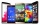Victoria's cell phone plan costs \$30.00 a month. If she used 12.5 hours in May, how much did Victoria pay per minute?
• ProveProve that k1 and k2 is the equations of two circles. Find the equation of the line that passes through the centers of these circles. k1: x2+y2+2x+4y+1=0 k2: x2+y2-8x+6y+9=0
• Investment1000\$ is invested at 10% compound interest. What factor is the capital multiplied by each year? How much will be there after n=12 years?
• Roses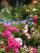On the large rosary was a third white, half red, yellow quarter and six pink. How many roses was in the rosary?
• Triangular prismCalculate the surface of a regular triangular prism with a bottom edge 8 of a length of 5 meters and an appropriate height of 60 meters and prism height is 1 whole 4 meters.
• Cuboid - ratiosThe sizes of the edges of the cuboid are in the ratio 2: 3: 5. The smallest wall have area 54 cm2. Calculate the surface area and volume of this cuboid.
• Two numbers 6Fill two natural numbers a, b: 7 + blank- blank = 5
• Odd/even numberPick any number. If that number is even, divide it by 2. If it's odd, multiply it by 3 and add 1. Now repeat the process with your new number. If you keep going, you'll eventually end up at 1. Every time. Prove. ..

Do you have an interesting mathematical word problem that you can't solve it? Submit a math problem, and we can try to solve it.

We will send a solution to your e-mail address. Solved examples are also published here. Please enter the e-mail correctly and check whether you don't have a full mailbox.

Please do not submit problems from current active competitions such as Mathematical Olympiad, correspondence seminars etc...